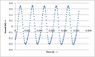# Sound wave regression

• oxnume

#### oxnume

We did a lab that involved using sound probes that gave us a bunch of data points which we were able to plot into a waveform using excel. However, we have to determine the equation of the waveform and it is expected that we include the uncertainty of the coefficients (much like using the LINEST command in excel) so is there a way to do trig regression in excel or maybe MAPLE (which I already have but don't know how to use)?

#### Attachments

•graph.png
4.9 KB · Views: 315
Last edited: